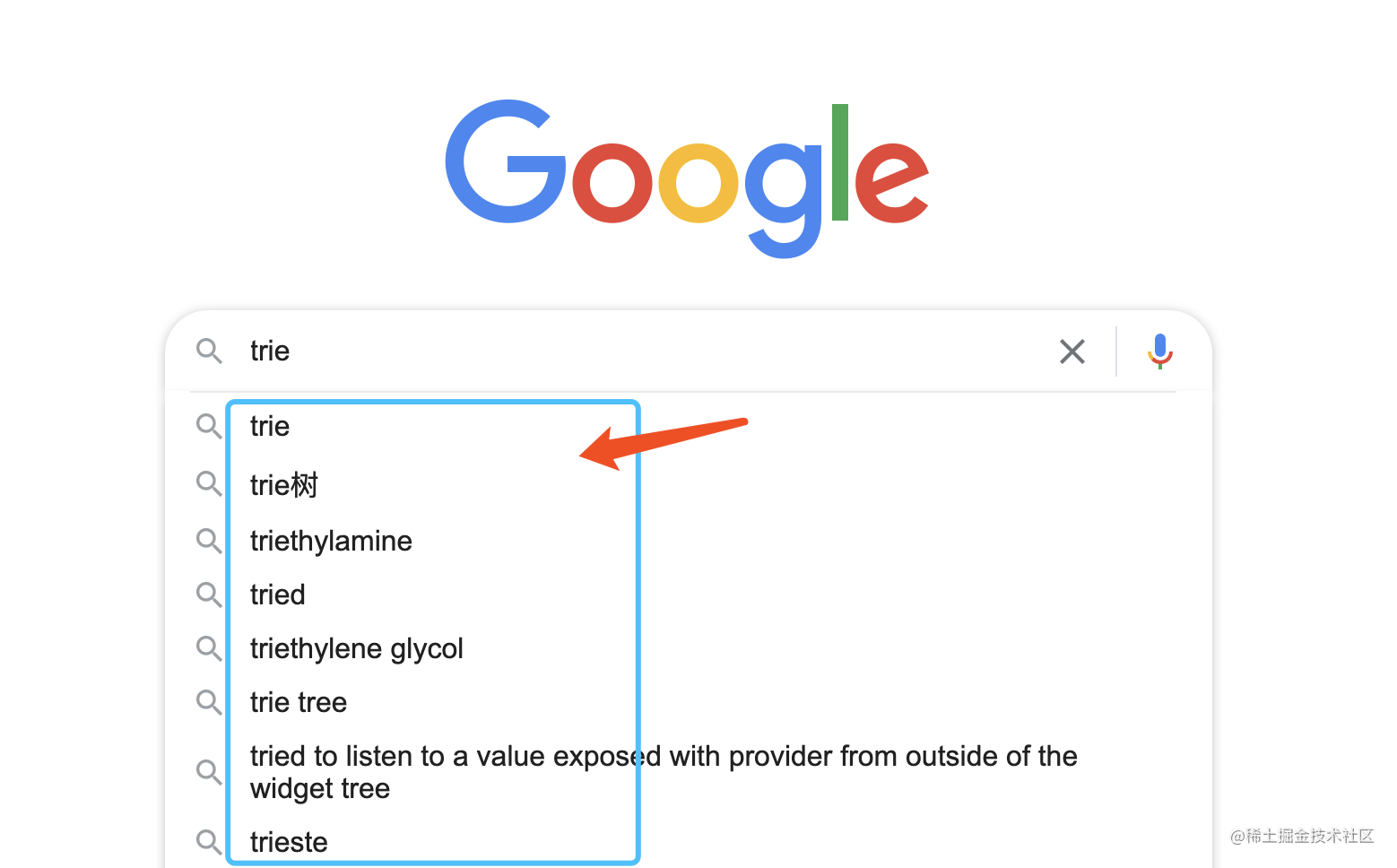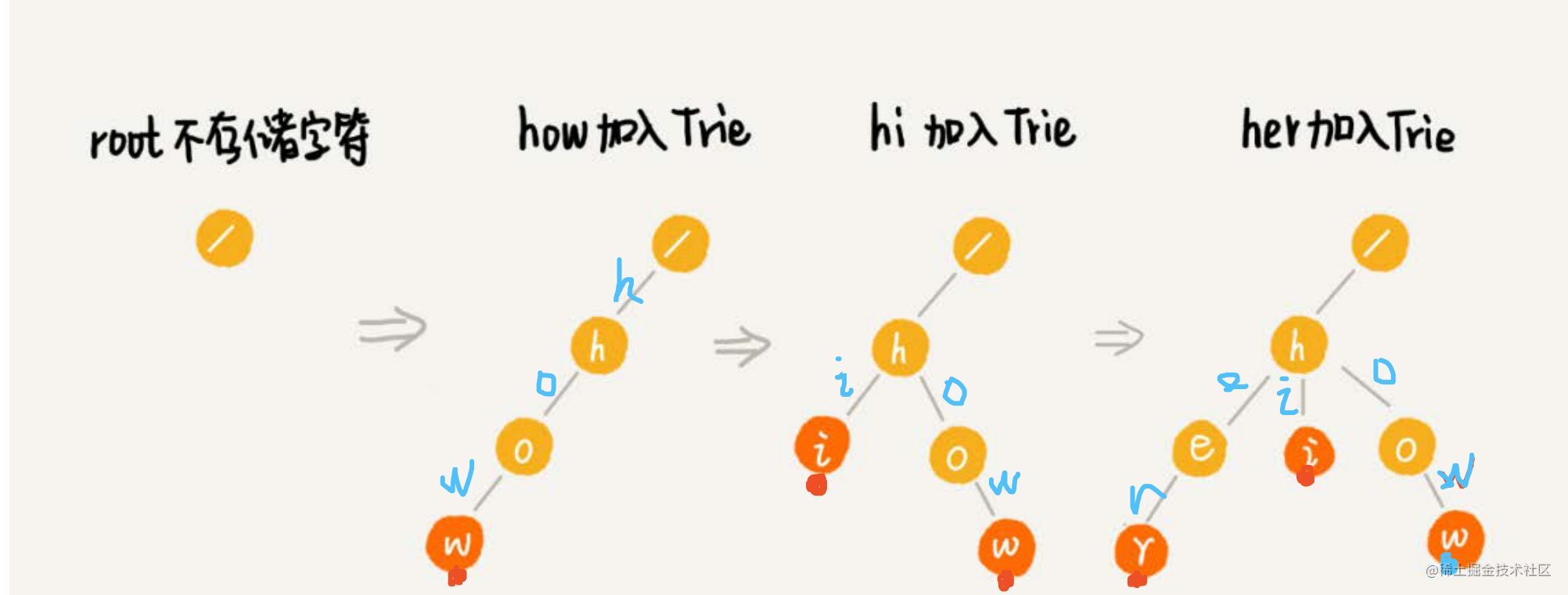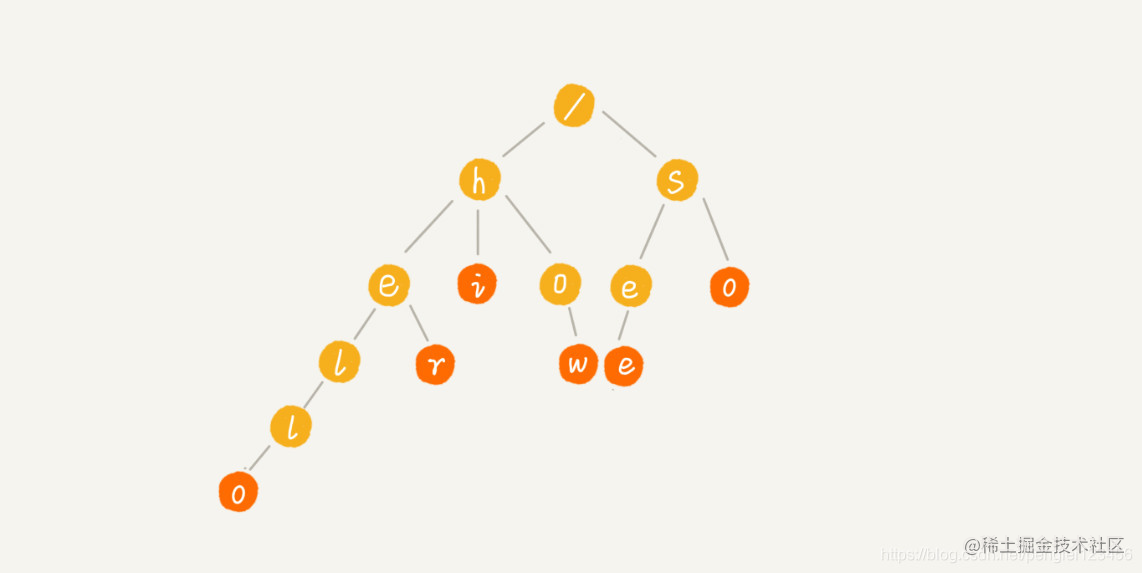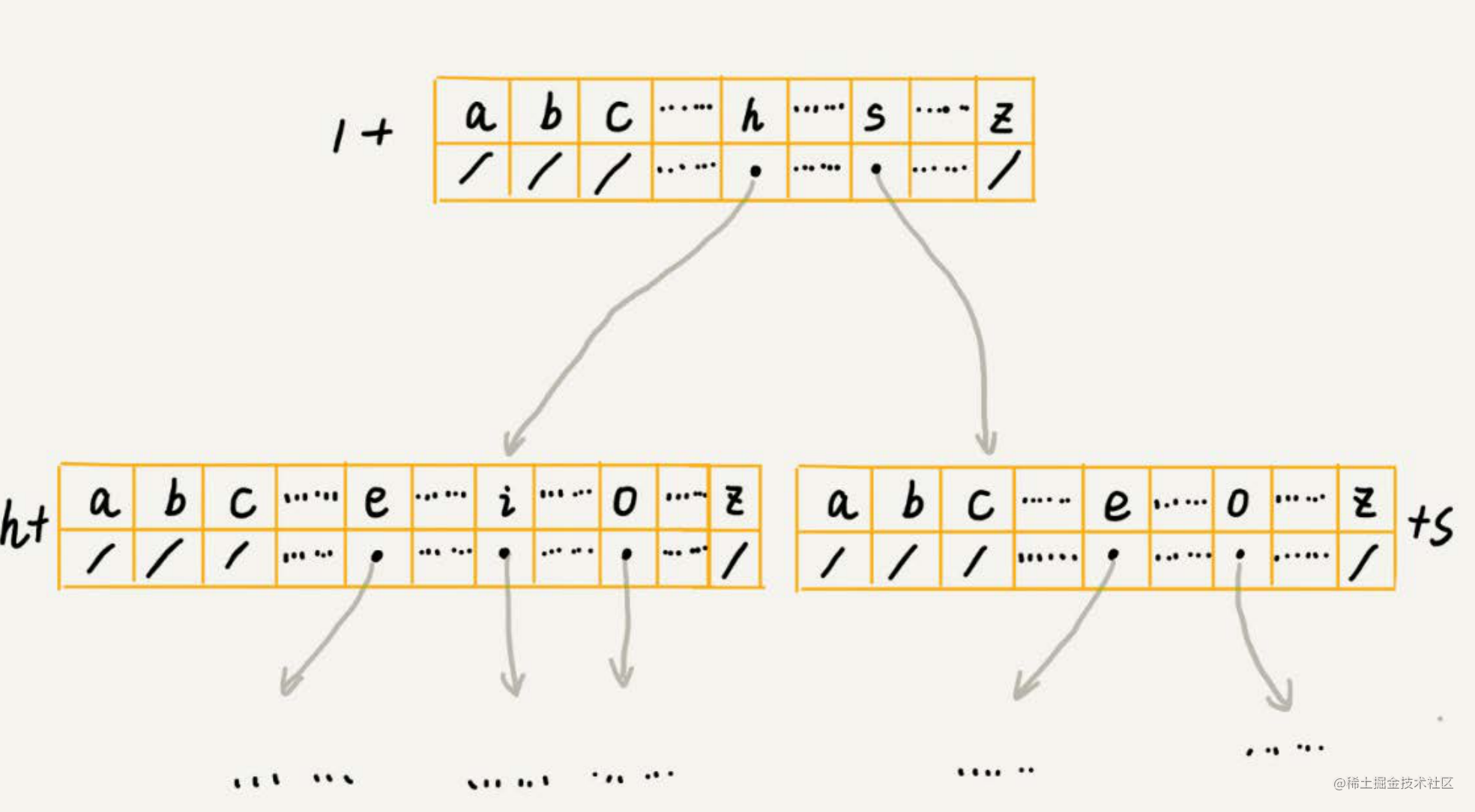# 前端算法必刷题系列

## 141. 实现 Trie (前缀树/字典树) (implement-trie-prefix-tree)

• Trie 前缀树/字典树
• 中等

### 题目

leetcode 传送门

`Trie`（发音类似 "try"）或者说 `前缀树` 是一种树形数据结构，用于高效地存储和检索字符串数据集中的键。这一数据结构有相当多的应用情景，例如`自动补完和拼写检查`

• `Trie()` 初始化前缀树对象。
• void `insert(String word)` 向前缀树中插入字符串 word 。
• boolean `search(String word)` 如果字符串 word 在前缀树中，返回 true（即，在检索之前已经插入）；否则，返回 false 。
• boolean `startsWith(String prefix)` 如果之前已经插入的字符串 word 的前缀之一为 prefix ，返回 true ；否则，返回 false 。

``````输入
["Trie", "insert", "search", "search", "startsWith", "insert", "search"]
[[], ["apple"], ["apple"], ["app"], ["app"], ["app"], ["app"]]

[null, null, true, false, true, null, true]

Trie trie = new Trie();
trie.insert("apple");
trie.search("apple");   // 返回 True
trie.search("app");     // 返回 False
trie.startsWith("app"); // 返回 True
trie.insert("app");
trie.search("app");     // 返回 True

### 基本思路

Tire 树也叫字典树。它是一个树形结构，专门用来处理字符串匹配的数据结构，用来解决在一组字符串集合中快速查找某个字符串的问题。例如搜索引擎的提示`how，hi，her，hello，so，see` 作为数据集建立 trie 树### 写法实现

``````var Trie = function() {
this.children = {}
};

/**
* Inserts a word into the trie.
* @param {string} word
* @return {void}
*/
Trie.prototype.insert = function(word) {
let node = this.children;
for (const ch of word) {
if (!node[ch]) {
node[ch] = {};
}
node = node[ch];
}
node.isEnd = true;
};

Trie.prototype.searchPrefix = function(prefix) {
let node = this.children
for (ch of prefix) {
if (!node[ch]) {
return false
}
node = node[ch]
}
return node
};

/**
* Returns if the word is in the trie.
* @param {string} word
* @return {boolean}
*/
Trie.prototype.search = function(word) {
let node = this.searchPrefix(word)
return node !== undefined && node.isEnd !== undefined
};

Trie.prototype.startsWith = function(prefix) {
return Boolean(this.searchPrefix(prefix))
};

// 测试
let trie = new Trie();
trie.insert("apple");
// 我们此时打印的话, 看看insert 之后 trie 结构, 这样就很清晰了
// console.log(trie)
// {
//     "children":{
//         "a":{
//             "p":{
//                 "p":{
//                     "l":{
//                         "e":{
//                             "isEnd":true
//                         }
//                     }
//                 }
//             }
//         }
//     }
// }
console.log(trie.search("apple"))
// 返回 true, 能查到，且isEnd 为 true 说明到末尾了
console.log(trie.search("app"))
// 返回 false，因为没到末尾，所以只能说匹配到前缀而不是单词
console.log(trie.startsWith("app"))
// 这次找到前缀就行

trie.insert("app");
// console.log(trie)
// {
//     "children":{
//         "a":{
//             "p":{
//                 "p":{
//                     "l":{
//                         "e":{
//                             "isEnd":true
//                         }
//                     },
//                     "isEnd":true  // 这里也有一个 app 的单词结尾了
//                 }
//             }
//         }
//     }
// }

console.log(trie.search("app"))
// true